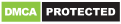### Write Comment

Type in
(Press Ctrl+g to toggle between English and the chosen language)

•The given statement can be written as $Hleq K geq D = B$ The conclusions can be written as (i) $H leq B$ (ii) $B leq K$ From the statememnt , it is clear that no relation can be established between H and B. Hence, conclusion (i) is false. We can see that conclusion (ii) is true. Hence, option B is the right answer.Powered By:Omega Web Solutions